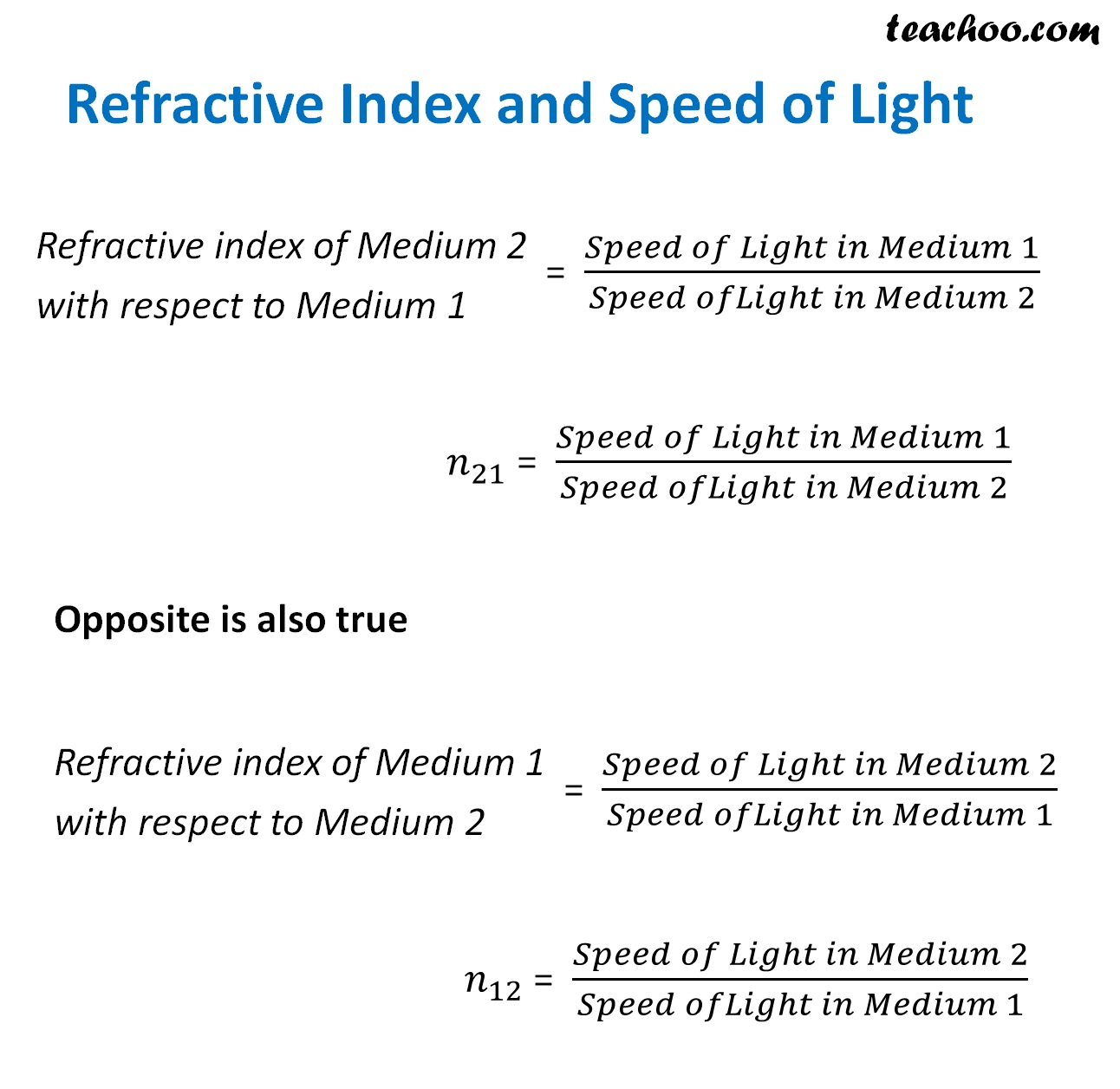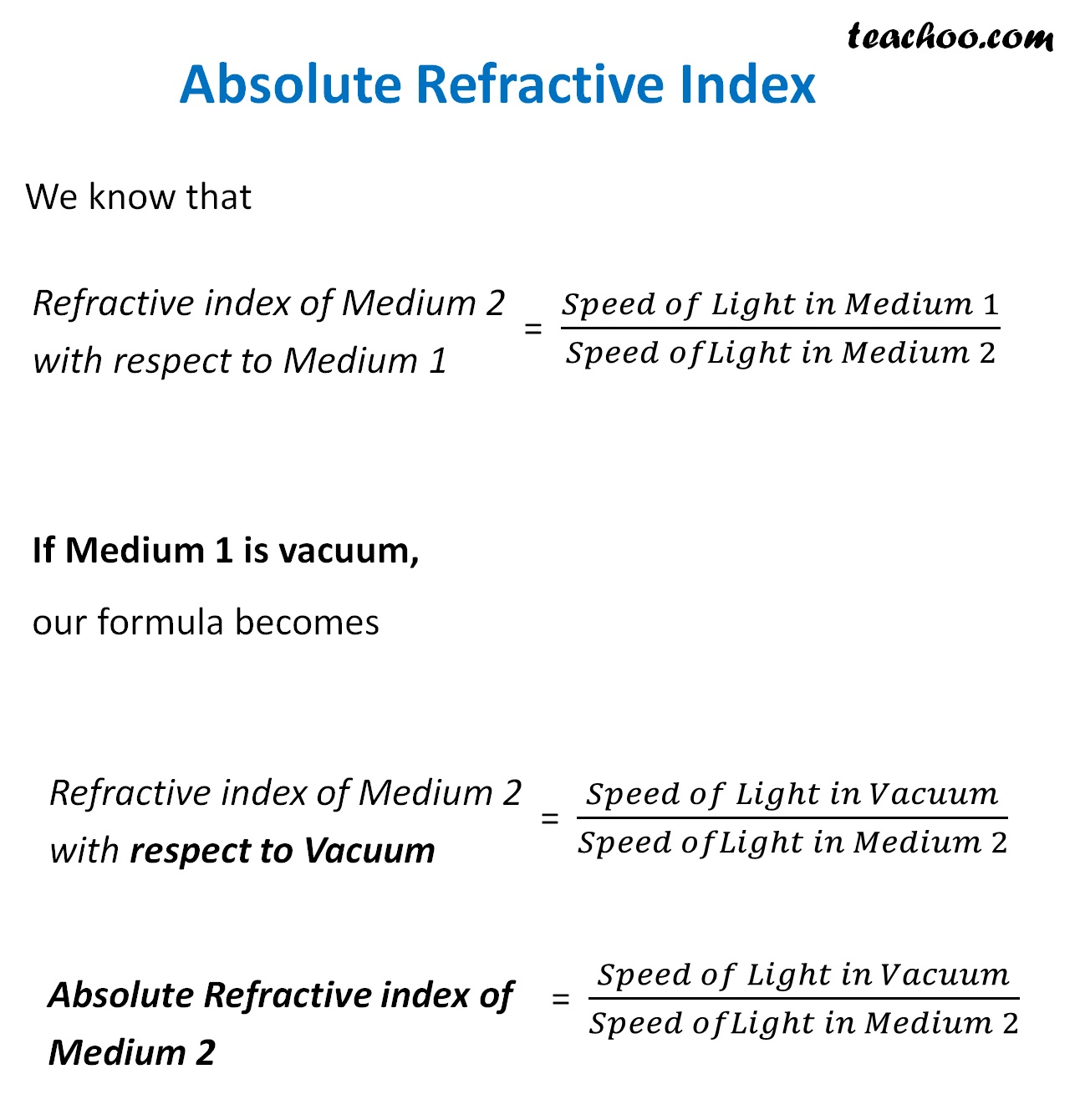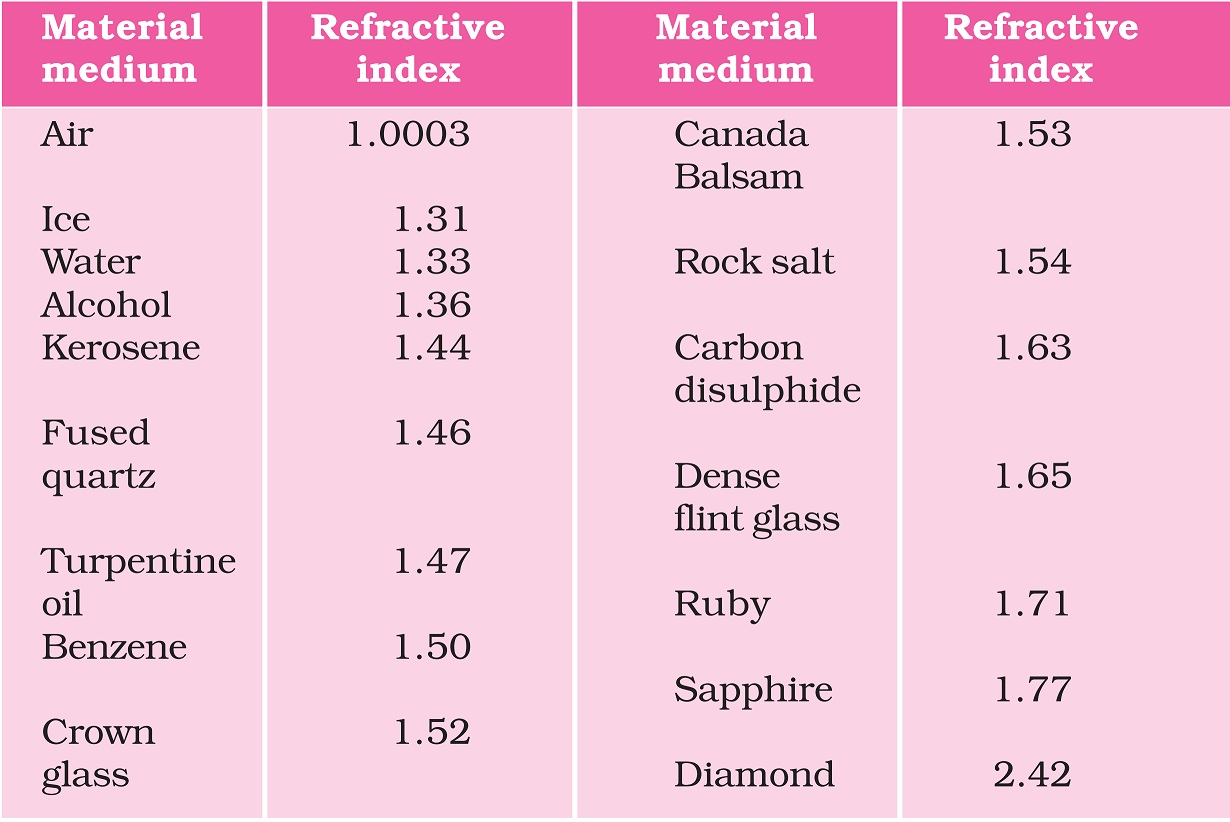Concepts

Class 10
Chapter 10 Class 10 - Light - Reflection and Refraction

We saw that Refractive Index is

the ratio of sin i and sin r

But, there is another formula of Refractive Index

## Relation of Refractive Index to Speed of Light

We know that light travels at different speeds in different mediums

It travels faster in air than in solids like glass

Refractive index means how slow light is travelling in 1 medium, compared to another

So, here.. we have two mediums (Example - air and glass), and refractive index is with respect to another medium## What is Absolute Refractive Index

The Refractive Index of a Medium with respect to vacuum is called Absolute Refractive Index## Absolute Refractive Index of Different Materials## What is Optically Rarer and Denser Medium

If we compare refractive index of 2 mediums

Those Mediums which have Higher Refractive Index are called Optically Denser Mediums

Those mediums which have a lower refractive index are called Optically Rarer Mediums

### Example

Refractive Index of Air is 1.0003 and Water is 1.33

It means that Water is Optically Denser Medium as compared to Air

We can also say that

Air is Optically Rarer Medium as compared to Water

## Important Points

If a ray of light travels from Optically Rarer Medium to a Denser Medium

It bends towards the normal

If a ray of light travels from Optically Denser Medium to a Rarer Medium

It bends away from normal

## What is Optical Density

The ability of a medium to refract light is called Optical Density

It depends upon Refractive Index of a Medium

If Medium has a higher Refractive Index, it means it has Higher Optical Density

## Questions

Question 2 Page 176 - Light enters from air to glass having refractive index 1.50. What is the speed of light in the glass? The speed of light in vacuum is 3 × 10 8 m s –1

Question 3 Page 176 - Find out, from Table 10.3, the medium having highest optical density. Also find the medium with lowest optical density

Question 4 Page 176 - You are given kerosene, turpentine and water. In which of these does the light travel fastest? Use the information given in Table 10.3

Question 5 Page 176 - The refractive index of diamond is 2.42. What is the meaning of this statement?

Learn in your speed, with individual attention - Teachoo Maths 1-on-1 Class

### Transcript

Refractive Index and Speed of Light Refractive index of Medium 2 with respect to Medium 1 = (𝑆𝑝𝑒𝑒𝑑 𝑜𝑓 𝐿𝑖𝑔ℎ𝑡 𝑖𝑛 𝑀𝑒𝑑𝑖𝑢𝑚 1)/(𝑆𝑝𝑒𝑒𝑑 𝑜𝑓𝐿𝑖𝑔ℎ𝑡 𝑖𝑛 𝑀𝑒𝑑𝑖𝑢𝑚 2) 𝑛_21 = (𝑆𝑝𝑒𝑒𝑑 𝑜𝑓 𝐿𝑖𝑔ℎ𝑡 𝑖𝑛 𝑀𝑒𝑑𝑖𝑢𝑚 1)/(𝑆𝑝𝑒𝑒𝑑 𝑜𝑓𝐿𝑖𝑔ℎ𝑡 𝑖𝑛 𝑀𝑒𝑑𝑖𝑢𝑚 2) Opposite is also true Refractive index of Medium 1 with respect to Medium 2 = (𝑆𝑝𝑒𝑒𝑑 𝑜𝑓 𝐿𝑖𝑔ℎ𝑡 𝑖𝑛 𝑀𝑒𝑑𝑖𝑢𝑚 2)/(𝑆𝑝𝑒𝑒𝑑 𝑜𝑓𝐿𝑖𝑔ℎ𝑡 𝑖𝑛 𝑀𝑒𝑑𝑖𝑢𝑚 1) 𝑛_12 = (𝑆𝑝𝑒𝑒𝑑 𝑜𝑓 𝐿𝑖𝑔ℎ𝑡 𝑖𝑛 𝑀𝑒𝑑𝑖𝑢𝑚 2)/(𝑆𝑝𝑒𝑒𝑑 𝑜𝑓𝐿𝑖𝑔ℎ𝑡 𝑖𝑛 𝑀𝑒𝑑𝑖𝑢𝑚 1) Absolute Refractive Index We know that Refractive index of Medium 2 with respect to Medium 1 = (𝑆𝑝𝑒𝑒𝑑 𝑜𝑓 𝐿𝑖𝑔ℎ𝑡 𝑖𝑛 𝑀𝑒𝑑𝑖𝑢𝑚 1)/(𝑆𝑝𝑒𝑒𝑑 𝑜𝑓𝐿𝑖𝑔ℎ𝑡 𝑖𝑛 𝑀𝑒𝑑𝑖𝑢𝑚 2) If Medium 1 is vacuum, our formula becomes Refractive index of Medium 2 with respect to Vacuum = (𝑆𝑝𝑒𝑒𝑑 𝑜𝑓 𝐿𝑖𝑔ℎ𝑡 𝑖𝑛 𝑉𝑎𝑐𝑢𝑢𝑚)/(𝑆𝑝𝑒𝑒𝑑 𝑜𝑓𝐿𝑖𝑔ℎ𝑡 𝑖𝑛 𝑀𝑒𝑑𝑖𝑢𝑚 2) Absolute Refractive index of Medium 2 = (𝑆𝑝𝑒𝑒𝑑 𝑜𝑓 𝐿𝑖𝑔ℎ𝑡 𝑖𝑛 𝑉𝑎𝑐𝑢𝑢𝑚)/(𝑆𝑝𝑒𝑒𝑑 𝑜𝑓𝐿𝑖𝑔ℎ𝑡 𝑖𝑛 𝑀𝑒𝑑𝑖𝑢𝑚 2)